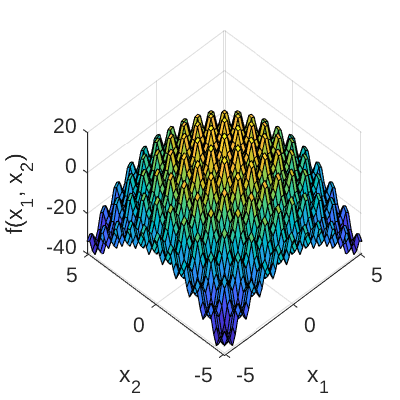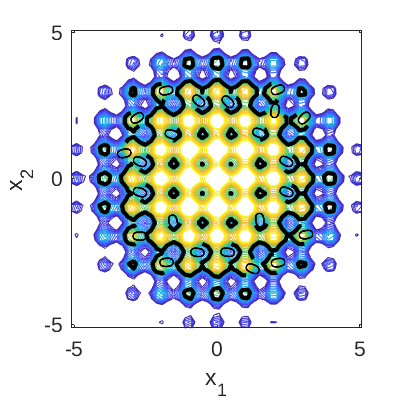# Modified Rastrigin Function

The 2-dimensional modified Rastrigin function is a test function for reliability analysis algorithms (Echard et al., 2011). It is a modification of the Rastrigin function (Mühlenbein et al. 1991) to include positive and negative values (i.e., safe and failed points, respectively). It features a highly non-linear limit state function with non-convex and non-connex failure domains (Echard et al., 2011).

## Description

The analytic expression of the modified Rastrigin function is given as:

g(\mathbf{x}) = 10 - \sum_{m=1}^2 (x_m^2 - 5 \cos{(2 \pi x_m)})

where \mathbf{x} = \{x_1, x_2\} is the vector of input variables.

The failure event and the failure probability are defined as g(\mathbf{x}) \leq 0 and P_f = \mathbb{P}[g(\mathbf{x}) \leq 0], respectively. Figure 1 and 2 show the surface and contour plots of the modified Rastrigin function, respectively. In Figure 2, the limit state function at which g(\mathbf{x}) = 0 is highlighted.Figure 1: Surface plot of the modified Rastrigin function.Figure 2: Contour plot of the modified Rastrigin function. The limit state function at which g(\mathbf{x}=0) is highlighted.

## Inputs

The inputs x_1 and x_2 are modeled as two independent standard Gaussian random variables.

No Variable Distribution Parameters
1 x_1 Gaussian \mu_{x_1} = 0,
\sigma_{x_1} = 1.0
2 x_2 Gaussian \mu_{x_2} = 0,
\sigma_{x_2} = 1.0

## Reference values

Some reference values for the failure probability P_f are given in the table below.

Method N \hat{P}_f \text{COV}[\hat{P}_f] Source
MCS 6 \times 10^4 7.34 \times 10^{-2} 1.5\% Echard et al. (2011)
MCS 10^6 7.31 \times 10^{-2} 0.36\% UQLab v1.2.1
Subset simulation 5'000 7.65 \times 10^{-2} - Echard et al. (2011)

## Resources

The vectorized implementation of the modified Rastrigin in MATLAB as well as the script file with the model and probabilistic inputs definitions for the function in UQLAB can be downloaded below:

uq_modifiedRastrigin.zip (2.4 KB)

The contents of the file are:

Filename Description
uq_modifiedRastrigin.m vectorized implementation of the modified Rastrigin function in MATLAB
uq_Example_modifiedRastrigin.m definitions for the model and probabilistic inputs in UQLab
LICENSE license for the function (BSD 3-Clause)

## References

• H. Mühlenbein, M. Schomisch, and J. Born, “The parallel genetic algorithm as function optimizer,” Parallel Computing, vol. 17, pp. 619–632, 1991. DOI:10.1016/S0167-8191(05)80052-3
• B. Echard, N. Gayton, and M. Lemaire, “AK-MCS: An active learning reliability method combining Kriging and Monte Carlo Simulation,” Structural Safety, vol. 33, pp. 145–154, 2011. DOI:10.1016/j.strusafe.2011.01.002